# Understanding Electricity

What is Electricity?

Any appliances that we use in our daily lives such as household appliances, office equipments and industrial equipments, almost all of those things take electricity. Therefore, we should understand electricity.

The first question that we will find out the answer is "where does electricity come from?"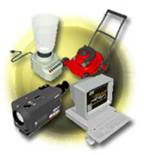All matters are made up of atoms. Then ask the next question, "What are atoms?"
Atoms are the smallest part of an element. They are composed of nucleus and electrons, electrons surround nucleus. Elements are identified by the number of electrons in orbit around nucleus of atoms and by the number of protons in nucleus.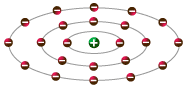Nucleus is made up of protons and neutrons, and the number of protons and neutrons are balanced. Neutrons have no electric charge, protons have positive charges (+) and electrons have negative charges (-). A positive charge of proton equals a negative charge of electron.

Electrons are bound in their orbit by attraction of protons, but electrons in the outer band can become free of their orbit by some external forces. These are referred to as free electrons, which move from one atom to the next, electron flows are produced. These are the basis of electricity. Materials that allow many electrons to move freely are called conductors and materials that allow few free electrons to move are called insulators.

All matters are made up of atoms that have electric charges. Therefore, they have electric charges. For the matter that has a balanced the number of protons and electrons, positive charge force and negative charge force are balanced. It is called neutral state of an atom. (The number of protons and electrons remains equal.)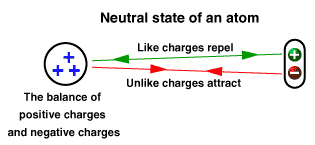"Static electricity" represents a situation that all things are made up of electric charges. For example, the rubbing of material against another can cause the static electricity. Free electrons of one material move forcefully till they are freed of their orbits around nucleus and move to another. Electrons of one material decrease, it presents positive charges. At the same time, electrons of another increase, it has negative charges.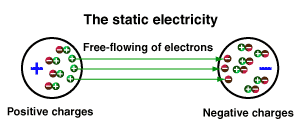In general, charge producing of the matter means the matter has electric charges. It has positive and negative charges, which is expressed in coulomb.

Current, Voltage and Resistance

What is Current?

An electrical phenomenon is caused by flow of free electrons from one atom to another. The characteristics of current electricity are opposite to those of static electricity.

Wires are made up of conductors such as copper or aluminum. Atoms of metal are made up of free electrons, which freely move from one atom to the next. If an electron is added in wire, a free electron is attracted to a proton to be neutral. Forcing electrons out of their orbits can cause a lack of electrons. Electrons, which continuously move in wire, are called Electric Current.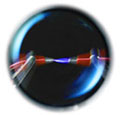For solid conductors
, electric current refers to directional negative-to-positive electrons from one atom to the next. Liquid conductors and gas conductors, electric current refers to electrons and protons flow in the opposite direction.

Current is flow of electrons, but current and electron flow in the opposite direction. Current flows from positive to negative and electron flows from negative to positive.

Current is determined by the number of electrons passing through a cross-section of a conductor in one second. Current is measured in amperes, which is abbreviated "amps". The symbol for amps is a letter "A".

A current of one amp means that current pass through a cross-section of two conductors, which are placed in parallel 1 meter apart with 2x10-7 Newton per meter force occur in each conductor. It can also mean charges of one coulomb (or 6.24x1018 electrons) passing through a cross-section of a conductor in one second.

What is voltage?

Electric current is flow of electrons in a conductor. The force required to make current flow through a conductor is called voltage and potential is the other term of voltage. For example, the first element has more positive charges, so it has higher potential. On the other hand, the second element has charges that are more negative so it has lower potential. The difference between two points is called potential difference.

Electromotive force means the force which makes current continuously flows through a conductor. This force can be generated from power generator, battery, flashlight battery and fuel cell, etc.

Volt, abbreviated "V", is the unit of measurement used interchangeably for voltage, potential, and electromotive force. One volt means a force which makes current of one amp move through a resistance of one ohm.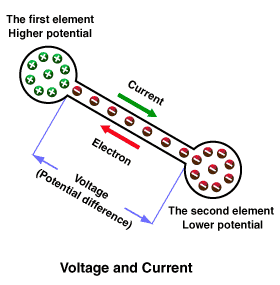What is resistance?
Electrons move through a conductor when electric current flows. All materials impede flow of electric current to some extent. This characteristic is called resistance. Resistance increases with an increase of length or decrease of cross-section of a material.

The unit of measurement for resistance is ohms and its symbol is the Greek letter omega (Ω). The resistance of one ohm means a conductor allows a current of one amp to flow with a voltage of one volt.

All materials are difference in allowing electrons flow. Materials that allow many electrons to flow freely are called conductors such as copper, silver, aluminium, hydrochloric solution, sulphuric acid and saltwater. In contrast, materials which allow few electrons to flow are called insulators such as plastic, rubber, glass and dry paper. Another type of materials, semiconductors have characteristics of both conductors and insulators. They allow electrons to move while being able to control flow of electrons and examples are carbon, silicon and germanium, etc.

The resistance of conductor depends on two main factors as the followings:

 1 Types of material 2 Temperature of material

How to measure current

The instrument used to measure current is called ampere meter or ammeter.
 � Steps for current measurement Connect a small light bulb to a dry cell. Measure current that passes through light bulb by connecting positive terminal (+) of ammeter to negative terminal (-) of a dry cell (see figure)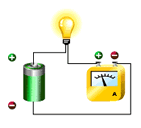� Safety instructions for current measurement; 1. Estimate current that required measuring then choose a suitable ammeter, since each ammeter has different limit of current measurement. 2. Be sure that the connection to positive terminal (+) and negative terminal (-) of ammeter are correct. 3. Do not directly connect ammeter terminals to dry cell terminals. Since it can damage the meter.

How to measure voltage
The instrument used to measure voltage, difference potential or electromotive force is called voltmeter.

 � Steps for voltage measurementConnect a small light bulb to a dry cell. A voltmeter is wired in parallel with the light bulb to measure voltage across the light bulb. Connect positive terminal (+) of voltmeter to positive terminal (+) of a dry cell and connect negative terminal (-) of voltmeter to negative terminal (-) of a dry cell (see figure).� Safety instructions for measuring voltage; 1. Estimate voltage that required measuring then choosea suitable voltmeter, since each voltmeter is designed withthe limit of voltage measurement. 2. Be sure that the connecting of positive terminal (+) and negative terminal (-) of voltmeter are correct.

How to measure resistance
The instrument used to measure resistance is called test meter or multimeter. The multimeter or test meter is used to make various electrical measurements such as current, voltage and resistance. It combines the functions of ammeter, voltmeter and ohmmeter.

 � Steps for resistance measurementTurn the face dial to a position for required measuring, resistance, then touch both of terminals of multimeter (see figure 1) and adjust the meter range to 0 Ω. Touch both of terminals of meter to a resistance and take the reading (see figure 2).Electric current is the ability to do work. Electric current can be converted to heat, power and magnetism, to name a few.

Electric current is classified by its functions and three primary types are:

 1 Heat and power 2 Electrochemistry 3 Magnetism

1. Heat and power is used to make heat and power.

 For example, current-carrying nichrom wire that nichrom wire has a high resistance and creates heat. This is applied to be component of electric ovens, toasters, electric irons and light bulbs, etc.Experiment is made by measuring heat quantity of water by calorimeter. Increase voltage across wire by the variac and connect ammeter and voltmeter to measure current and voltage.Set the variac scale to adjust voltage and current value of nichrom wire and current is passed through periodically and measure heat quantity from nichrom wire. There are any indications of voltage and current. If voltage, current and time increase, heat quantity will also increase. They are expressed by the relation as below.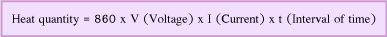This is called Joule's law. Heat quantity depends on voltage time current and interval of time. From Ohm's law, V (Voltage) = I (Current) x R (Resistance) therefore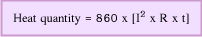Heat quantity depends on current squared times resistance and interval of time.When current is passed through nichrom wire in water, current is converted to heat and temperature rises. Work is done by heat generated in an electrical circuit, which is called Electric power.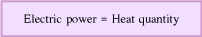Electric power is measured in Watts-hour (Wh) and heat quantity is measured in calories (Cal).Work is done by heat generated in an electrical circuit is written in power, which it means that the rate work is done in a circuit when 1 Amp flows with 1 Volt applied and its unit of measurement is Watt.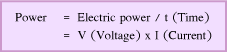Conclusion2. Electrochemistry

 For example, when current is passed through sodium chloride (NaCl) solution, a chemical reaction called electrolysis occurs. This is applied to produce electrolysis, galvanizing and battery, etc.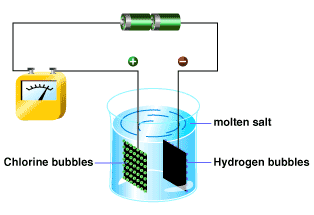Experiment is made by soaking two platinum (Pt) plates in molten salt. Connect batteries to two platinum plates, current is passed through molten salt and produces chlorine bubbles around positive plate (+) and hydrogen bubbles around negative plate (-) since sodium chloride composes of sodium (Na) and chloride (Cl). When sodium chloride melts in water, the elements are separated. Sodium has positive charges (+), while chlorine has negative charges (-) and these charges are called ions. The molten salt has both positive charges, called anodes, and negative charges called cathodes. The state of separated elements is called ionization. If salt is melted by water, solution is available ions, called electrolyte solution. And if current is passed through electrolyte solution, a chemical reaction known as electrolysis occurs.

3. Magnetism

 The example of this electric work is a current-carrying wire, magnetic lines of flux occur. This is applied to produce electric motors, electric transformers and tape recorders, etc.

Understanding meaning of magnetism:
What is magnetism?

The compound formula of magnet is Fe3O4. All magnets have two characteristics. First, they attract and hold iron. Secondary, if free to move like the compass needle, they will assume a north-south position. Any materials have these characteristics, they are called magnet.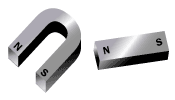The characteristics of magnet are � Every magnet has two poles, one north pole and one south pole. � Opposite poles attract each other, while like poles repel each other.
 Electricity and magnetic fieldWhen magnetic needle is placed near electric wire, which current is passed through, magnetic needle turns on the direction of current flow (see figure 1 and 2). Therefore, electric current flow also produces an associated magnetic force or it is said that electricity is able to produce magnetic field.When magnetic needle is placed in wire coil with one loop (see figure) and current is passed through wire coil, magnetic needle turns on the direction as shown in above figure. And the directions of magnetic lines of flux are shown by the arrows.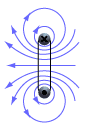When magnetic needle is placed in wire coil with many loops as shown in right figure, then current is passed through coil. The direction of magnetic lines of flux parallels wire coil. The characteristics of magnetic lines of flux like the characteristics of magnet, but no magnetic pole.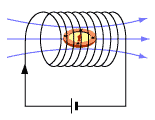When a current-carrying wire coil is placed near iron bar, the iron bar move slightly (see figure 1). If core is placed in a wire coil, the iron bar is attracted strongly (see figure 2). Because core is a soft iron, which conducts magnetic lines of force, when current is passed through wire coil around core, the core becomes magnetized with high power that is called electromagnets. This function is widely applied for using in industries.